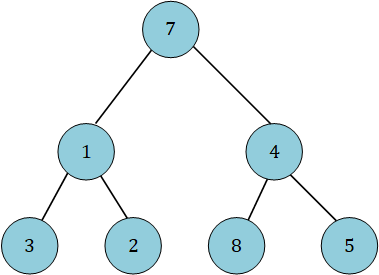New update is available. Click here to update.
Last Updated: 24 Mar, 2021
##### Lowest Common Ancestor of a Binary Tree III
Moderate
Problem statement#### Note:

``````Let ‘TREE’ be a binary tree. The lowest common ancestor of two nodes, ‘N1’ and ‘N2’, is defined as the lowest node in ‘TREE’ with ‘N1’ and ‘N2’ as descendants (where we allow a node to be a descendant of itself).
``````

#### Input Format:

``````The first line of input contains an integer 'T' representing the number of test cases.

The first line of each test case contains two single space-separated integers, ‘N1’ and ‘N2’, representing the nodes for which LCA has to be found.

The second line of each test case contains elements in the level order form. The line consists of values of nodes separated by a single space. In case a node is ‘NULL’, we take -1 in its place.
``````

#### For example:

``````The input for the tree depicted in the below image would be :
````````````7
1 4
3 2 8 5
-1 -1 -1 -1 -1 -1 -1 -1
``````

#### Explanation :

``````Level 1 :
The root node of the tree is 7

Level 2 :
Left child of 7 = 1
Right child of 7 = 4

Level 3 :
Left child of 1 = 3
Right child of 1 = 2
Left child of 4 = 8
Right child of 4 = 5

Level 4 :
Left child of 3 = NULL(-1)
Right child of 3 = NULL(-1)
Left child of 2 = NULL(-1)
Right child of 2 = NULL(-1)
Left child of 8 = NULL(-1)
Right child of 8 = NULL(-1)
Left child of 5 = NULL(-1)
Right child of 5 = NULL(-1)

The first not-null node(of the previous level) is treated as the parent of the first two nodes of the current level. The second not-null node (of the previous level) is treated as the parent node for the next two nodes of the current level and so on.
The input ends when all nodes at the last level are ‘NULL’(-1).
``````
##### Note :
``````The above format was just to provide clarity on how the input is formed for a given tree.

The sequence will be put together in a single line separated by a single space. Hence, for the above-depicted tree, the input will be given as:

7 1 4 3 2 8 5 -1 -1 -1 -1 -1 -1 -1 -1
``````
##### Output Format :
``````For each test case, print a single line containing a single integer denoting the LCA of given nodes N1 and N2.

The output for each test case is printed in a separate line.
``````

#### Note:

``````You do not need to print anything, it has already been taken care of. Just implement the given function.
``````
##### Constraints :
``````1 <= T <= 100
1 <= N <= 10 ^ 4
1 <= DATA <= 10 ^ 4
N1 != N2
N1 and N2 exist in the ‘TREE’.
The ‘TREE’ contains unique nodes.

Where ‘T’ is the number of test cases, ‘N’ is the number of nodes in the ‘TREE’, ‘DATA’ represents the value of the node, ‘N1’ and ‘N2’ represent the nodes of which LCA has to be found.

Time limit: 1 sec.
``````Approaches

## 01ApproachFor both the nodes, the idea is to use the ‘parent’ pointer to traverse up in the tree and keep track of their ancestors.

Firstly, for the node ‘n1’, we will traverse up in the tree till the root node and store all of its ancestors in a hash set. Then, we will traverse the tree using ‘n2’. While traversing, we will check whether ‘n2’ or any of its ancestors is present in the hash set. The first node to be found in the hash set will be LCA of ‘n1’ and ‘n2’.

Algorithm:

• Create a hash set, ‘hashSet’ to store the ancestors of node ‘n1’.
• Run a loop while ‘n1’ is not ‘NULL’.
• Insert the data of node ‘n1’ in the ‘hashSet’.
• Update ‘n1’ by its parent.
• Again run a loop while ‘n2’ is not ‘NULL’.
• If hashSet.count(n2->data) != 0 that shows that data of node ‘n2’ is already present in ‘hashSet’. So, return node ‘n2’.
• Else update ‘n2’ by its parent.